﻿ 多模态医学影像的非刚体配准与多分辨率融合方法
«上一篇文章快速检索 高级检索

 应用科技2019, Vol. 46Issue (3): 33-39  DOI: 10.11991/yykj.2018080070

### 引用本文ZHANG Xinyi, ZHANG Fuli, WANG Qiusheng. Method of non-rigid registration and multi-resolution fusion for multi-modal medical images[J]. Applied Science and Technology, 2019, 46(3), 33-39. DOI: 10.11991/yykj.201808007.### 文章历史

1. 北京航空航天大学 自动化科学与电气工程学院，北京 100191;
2. 中国人民解放军陆军总医院，北京 100700

Method of non-rigid registration and multi-resolution fusion for multi-modal medical images
ZHANG Xinyi 1, ZHANG Fuli 2 , WANG Qiusheng 1
1. School of Automation Science and Electrical Engineering, Beihang University, Beijing 100191, China;
2. PLA Army General Hospital, Beijing 100700, China
Abstract: In order to utilize effective information in medical images from different modalities, a special method for multi-modal medical image registration and image fusion is given in this paper. Firstly, to achieve the spatial geometric alignment of multi-modal medical images with large gray-scale and morphological difference, a high-performance registration method with mutual information measure is proposed, which is a combination of coarse registration based on affine transformation and fine registration based on B-spline transformation. Secondly, a multi-resolution fusion method based on wavelet transform is proposed to fuse wavelet coefficients of different frequency bands. The experimental results show that the proposed method can achieve the spatial alignment and the high-quality fusion of multi-modal medical images.
Keywords: multi-modal medical image    image registration    image fusion    mutual information measure    B-Spline transformation    affine transformation    wavelet transform    fusion rule

1 多模态医学影像非刚体配准方法 1.1 医学影像配准框架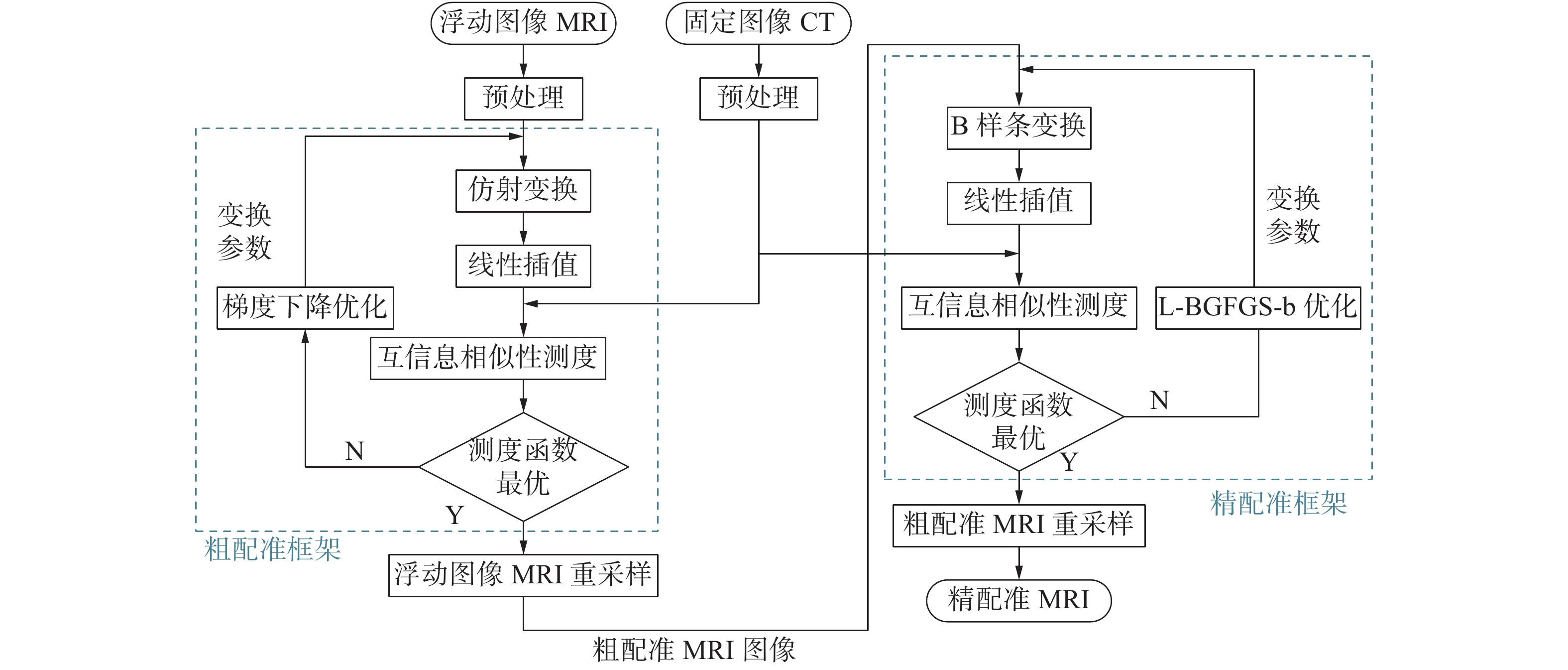Download: 图 1 配准流程
1.2 粗配准方法 1.2.1 多模态影像配准下相似性测度方法

 $\begin{array}{l} I\left( {A,B} \right) = H\left( B \right) - H\left( {B\left| A \right.} \right) = H\left( A \right) + H\left( B \right) - H\left( {A,B} \right) \end{array}$

 \left\{ \begin{aligned} & H\left( A \right) = - \sum\limits_a {{p_A}\left( a \right)\log {p_A}\left( a \right)} \\ & H\left( B \right) = - \sum\limits_b {{p_B}\left( b \right)\log {p_B}\left( b \right)} \\ & H\left( {A,B} \right) = - \sum\limits_{a,b} {{p_{A,B}}\left( {a,b} \right)\log {p_{A,B}}\left( {a,b} \right)} \end{aligned}\right.

1.2.2 粗配准变换方法

 $\left( {\begin{array}{*{20}{c}} {x'}\\ {y'}\\ {z'} \end{array}} \right) = \left( {\begin{array}{*{20}{c}} a&b&{{t_x}}\\ c&d&{{t_y}}\\ 0&0&1 \end{array}} \right)\left( {\begin{array}{*{20}{c}} x\\ y\\ 1 \end{array}} \right)$ (1)

1.2.3 粗配准结果分析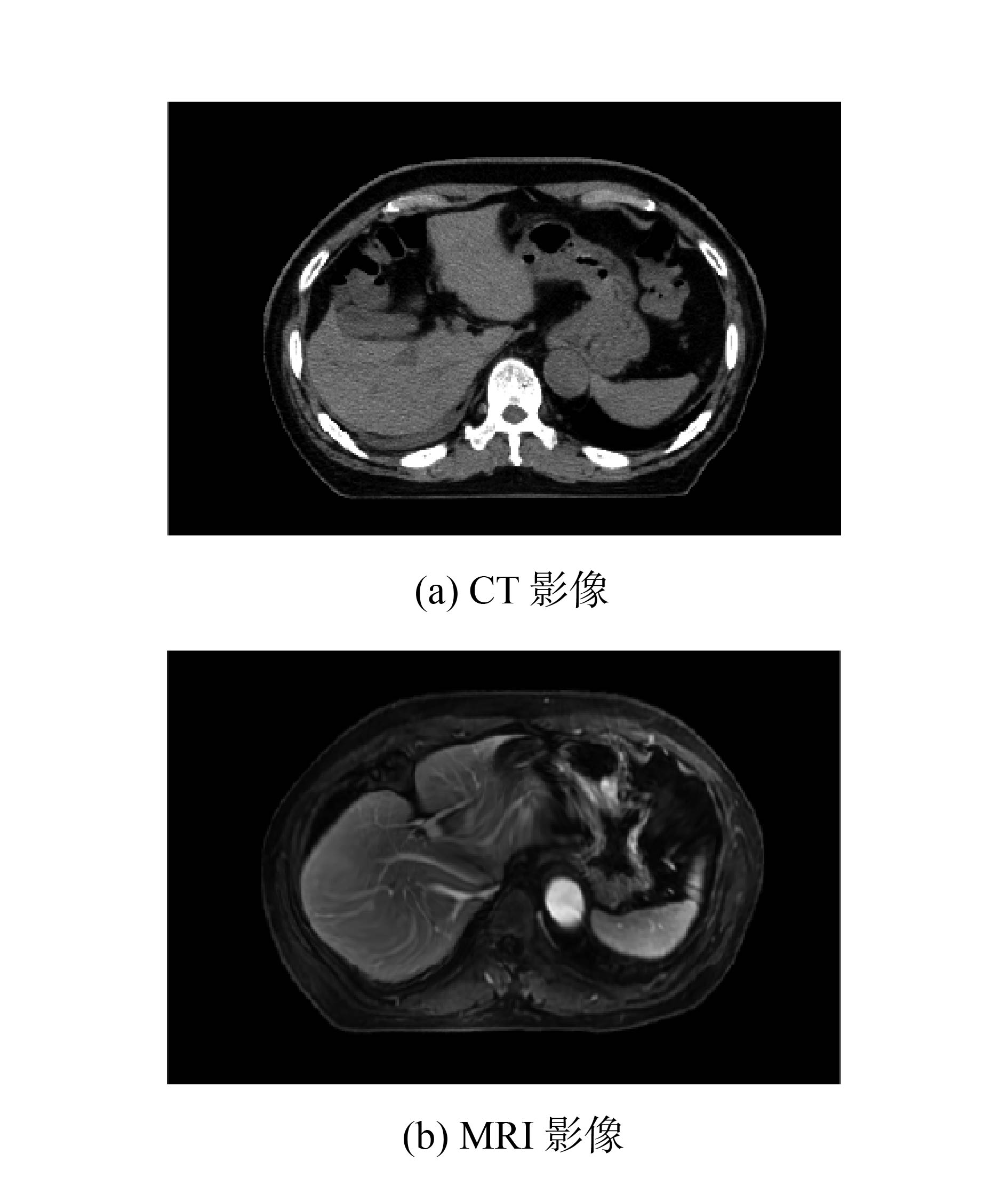Download: 图 2 原始影像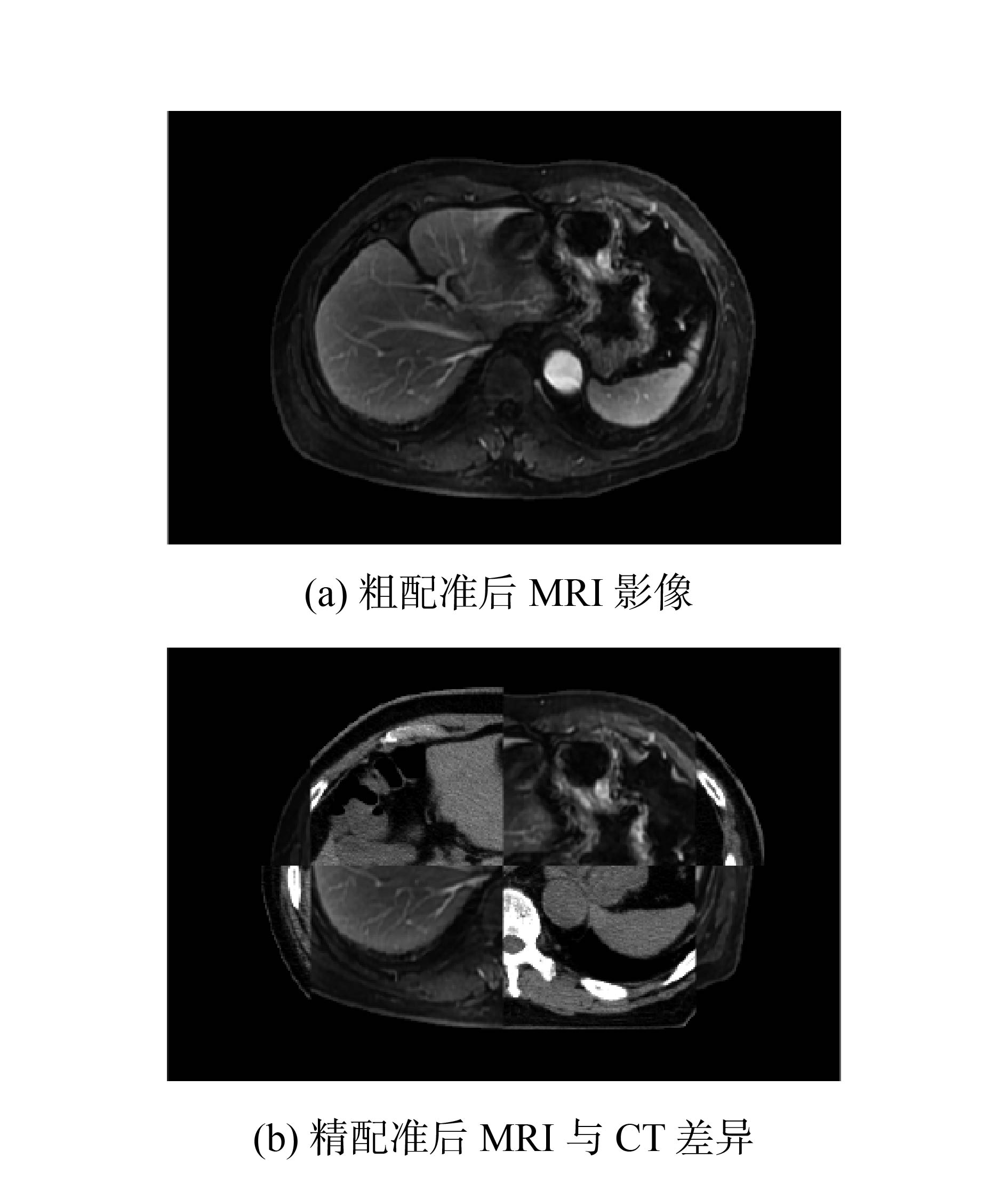Download: 图 3 粗配准结果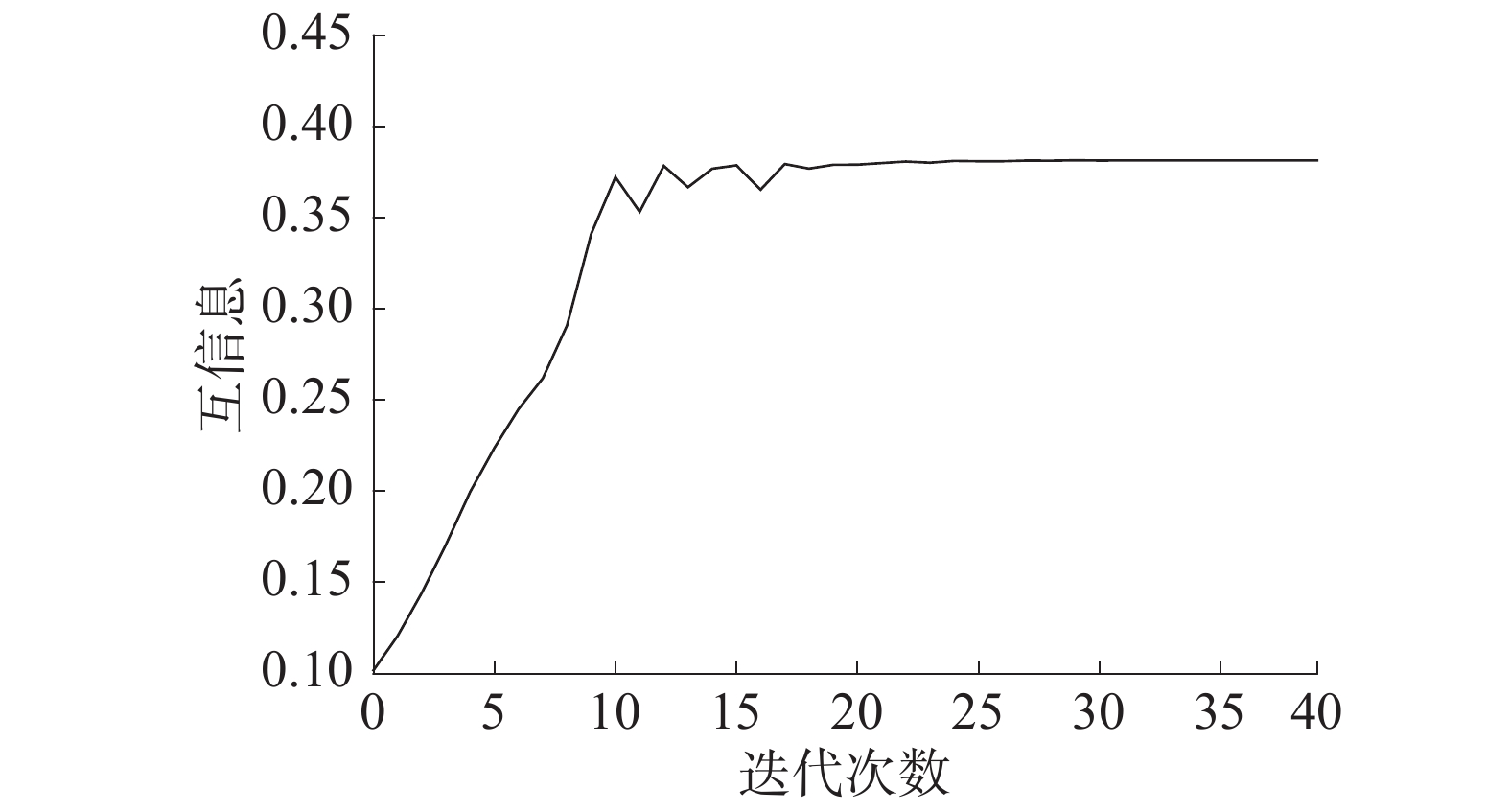Download: 图 4 粗配准互信息变化曲线
1.3 非刚体配准框架的方法 1.3.1 精配准框架中的变换方法

 $T(x,y,z) = \sum\limits_{l = 0}^3 {\sum\limits_{m = 0}^3 {\sum\limits_{n = 0}^3 {{B_l}(u){B_m}(v){B_n}(w){\phi _{i + l,j + m,k + n}}} } }$

 \left\{ \begin{aligned} & {B_0}(u) = {(1 - u)^3}/6\\ & {B_1}(u) = (3{u^3} - 6{u^2} + 4)/6\\ & {B_2}(u) = ( - 3{u^3} + 3{u^2} + 3u + 1)/6\\ & {B_3}(u) = {u^3}/6 \end{aligned}\right.

B样条模型的优点在于它对影像操作是局部的，即控制点位置变化只影响控制点邻域的变化，这样配准问题就等价于三次B样条的局部控制问题，从而使B样条计算效率更高，精度也能达到亚像素级

1.3.2 精配准结果分析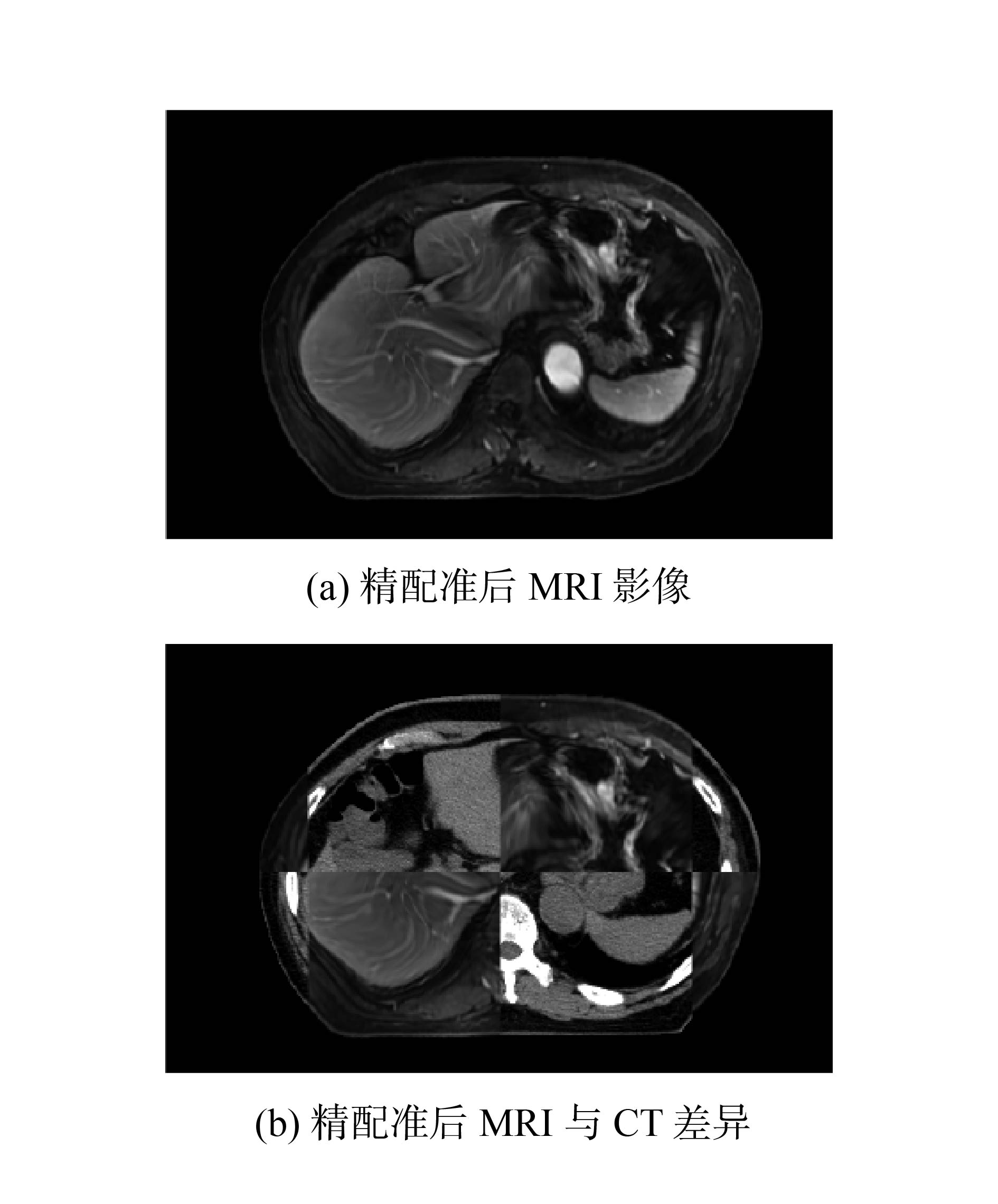Download: 图 5 精配准结果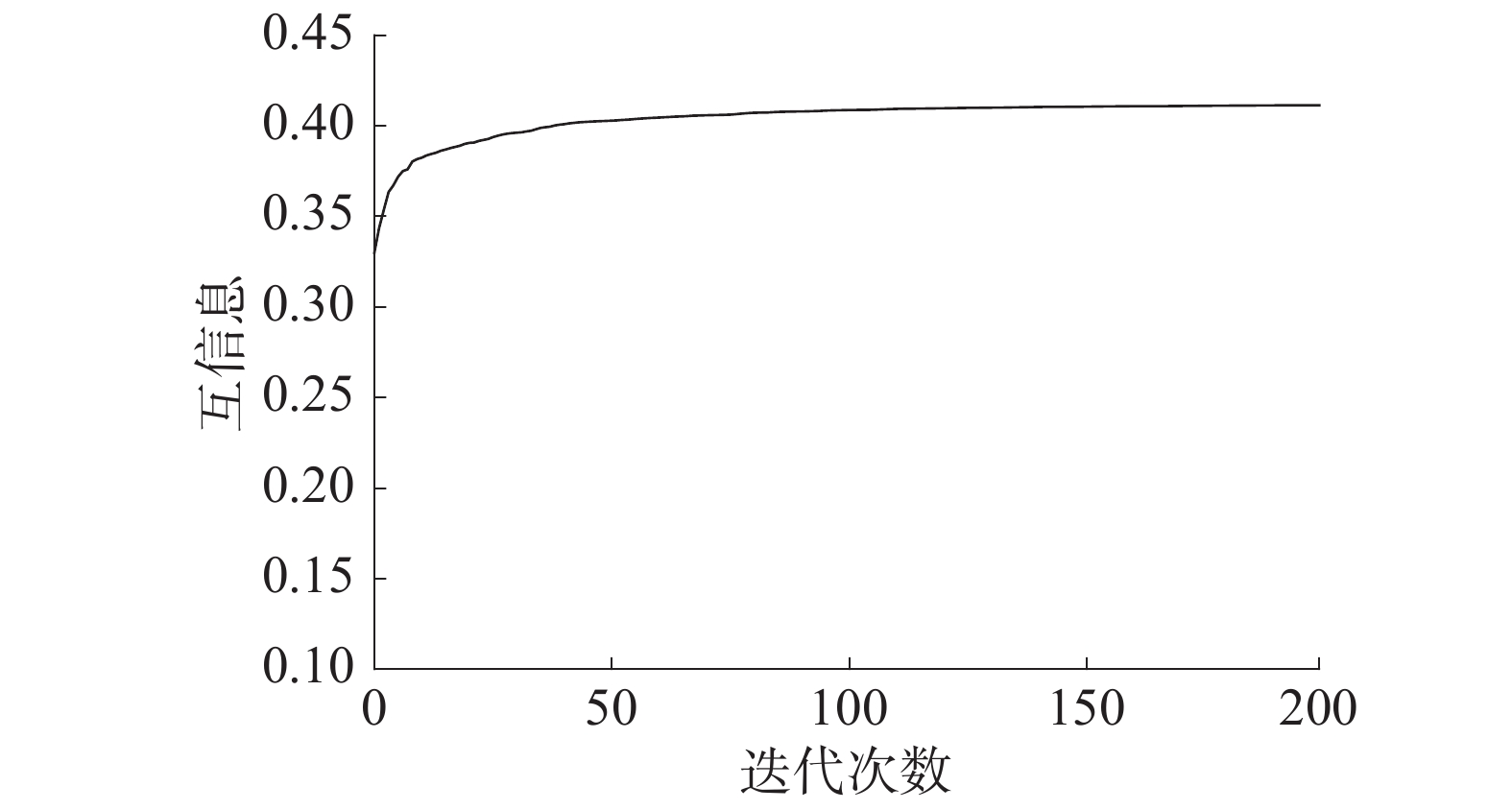Download: 图 6 精配准互信息变化曲线

2 多模态医学影像多分辨率融合方法

2.1 小波变换融合方法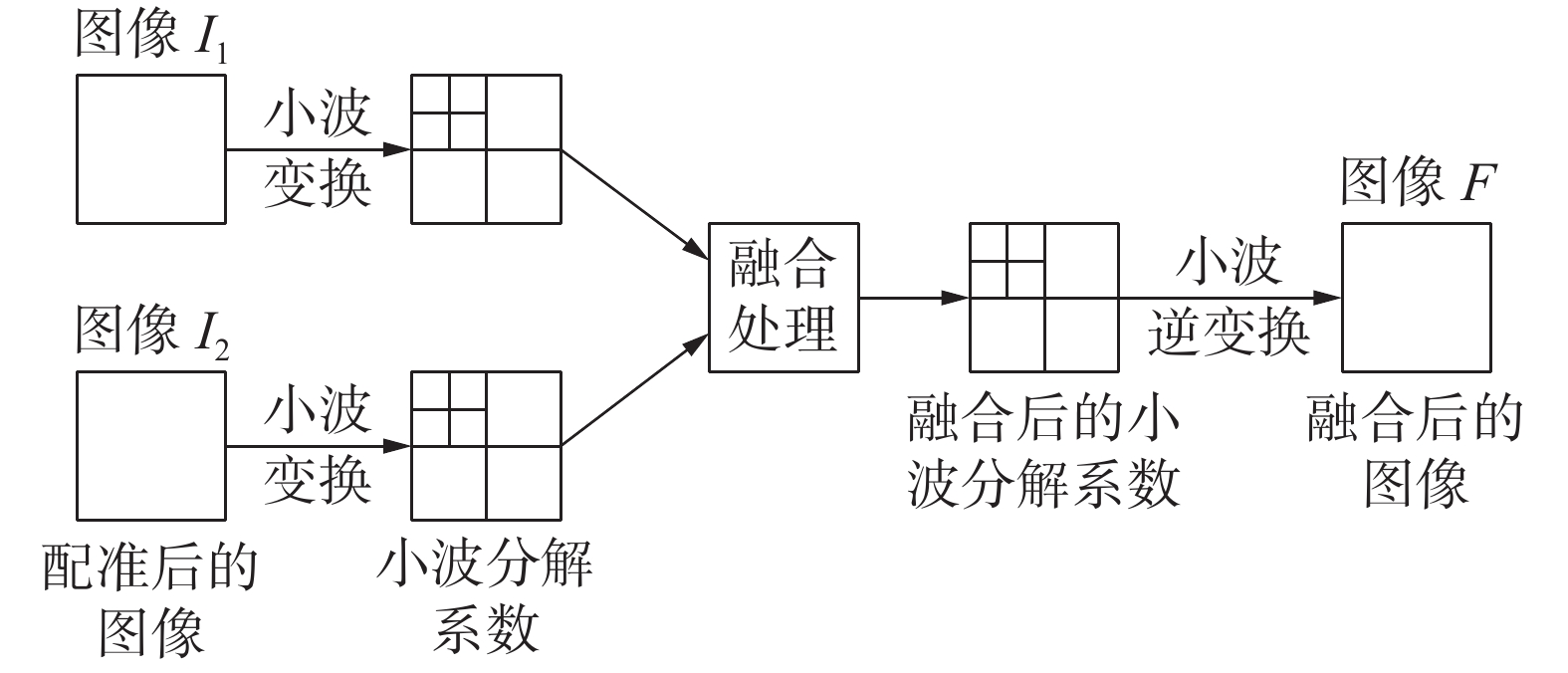Download: 图 7 小波融合过程示意

2.2 二维小波分解与重构

 \left\{ {\begin{aligned} & {{{C}_{j + 1}} = {L}{{C}_j}{{L}^{^*}}}\\ & {{D}_{j + 1}^H = {H}{{C}_j}{{L}^{^*}}}\\ & {{D}_{j + 1}^V = {L}{{C}_j}{{H}^{^*}}}\\ & {{D}_{j + 1}^D = {H}{{C}_j}{{H}^{^*}}} \end{aligned}} \right.\;\;\;\;\;\;j = 0,1, \cdots ,J - 1 (2)

 ${{C}_{j - 1}} = {{L}^{^*}}{{C}_j}{L} + {{H}^{^*}}{D}_j^H{L} + {{L}^{^*}}{D}_j^V{H}{\rm{ + }}{{H}^{^*}}{D}_j^D{H}$
2.3 小波变换融合规则

CT和MRI影像分解后不同频带系数反映的影像信息不同，低频系数融合规则侧重于反映多模影像的共同轮廓特征，高频系数融合规则侧重于反映尽可能多的细节信息。因此对不同频带小波系数需要选取不同的融合规则。

2.3.1 低频系数融合规则

 $D_F^j\left( {m,n} \right) = {c_1}D_A^j\left( {m,n} \right) + \left( {1 - {c_1}} \right)D_B^j\left( {m,n} \right)$ (3)

2.3.2 高频系数融合规则

1）绝对值最大法

 $D_F^j\left( {m,n} \right){\rm{ = }}\max \left( {\left| {D_A^j\left( {m,n} \right)} \right|,\left| {D_B^j\left( {m,n} \right)} \right|} \right)$ (4)

2） 区域方差最大法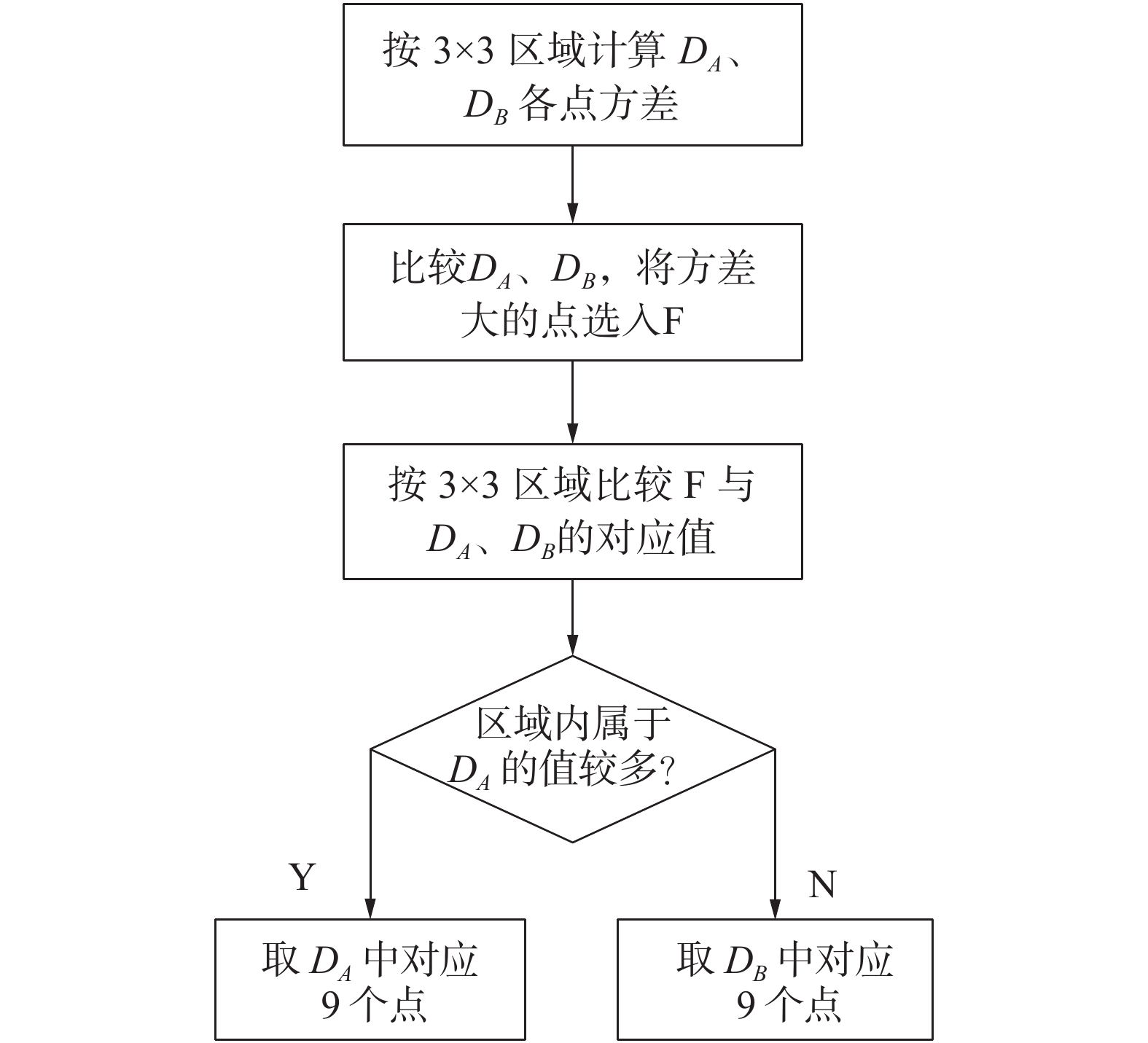Download: 图 8 区域方差最大法

3)区域能量融合法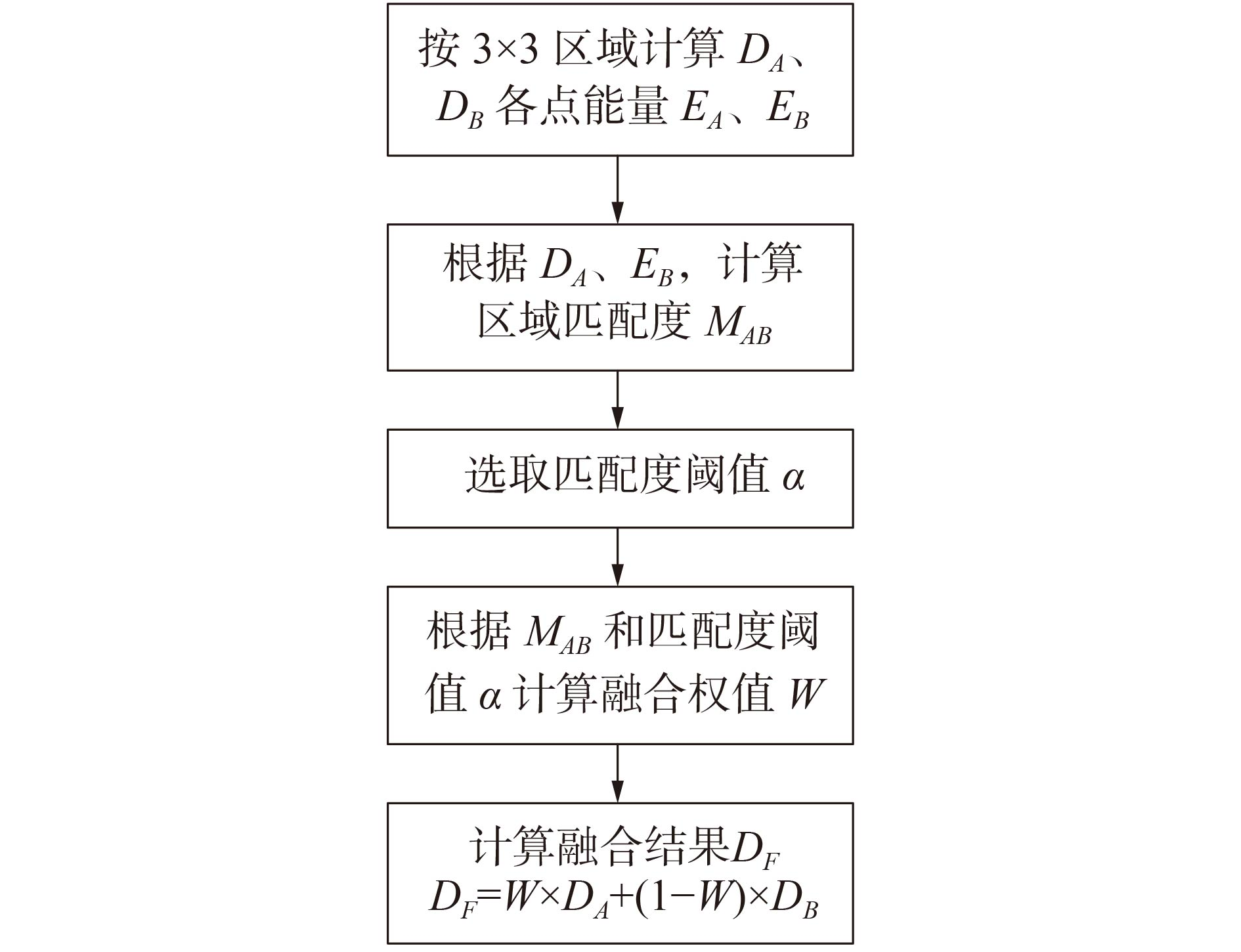Download: 图 9 区域能量融合法

2.4 影像融合结果分析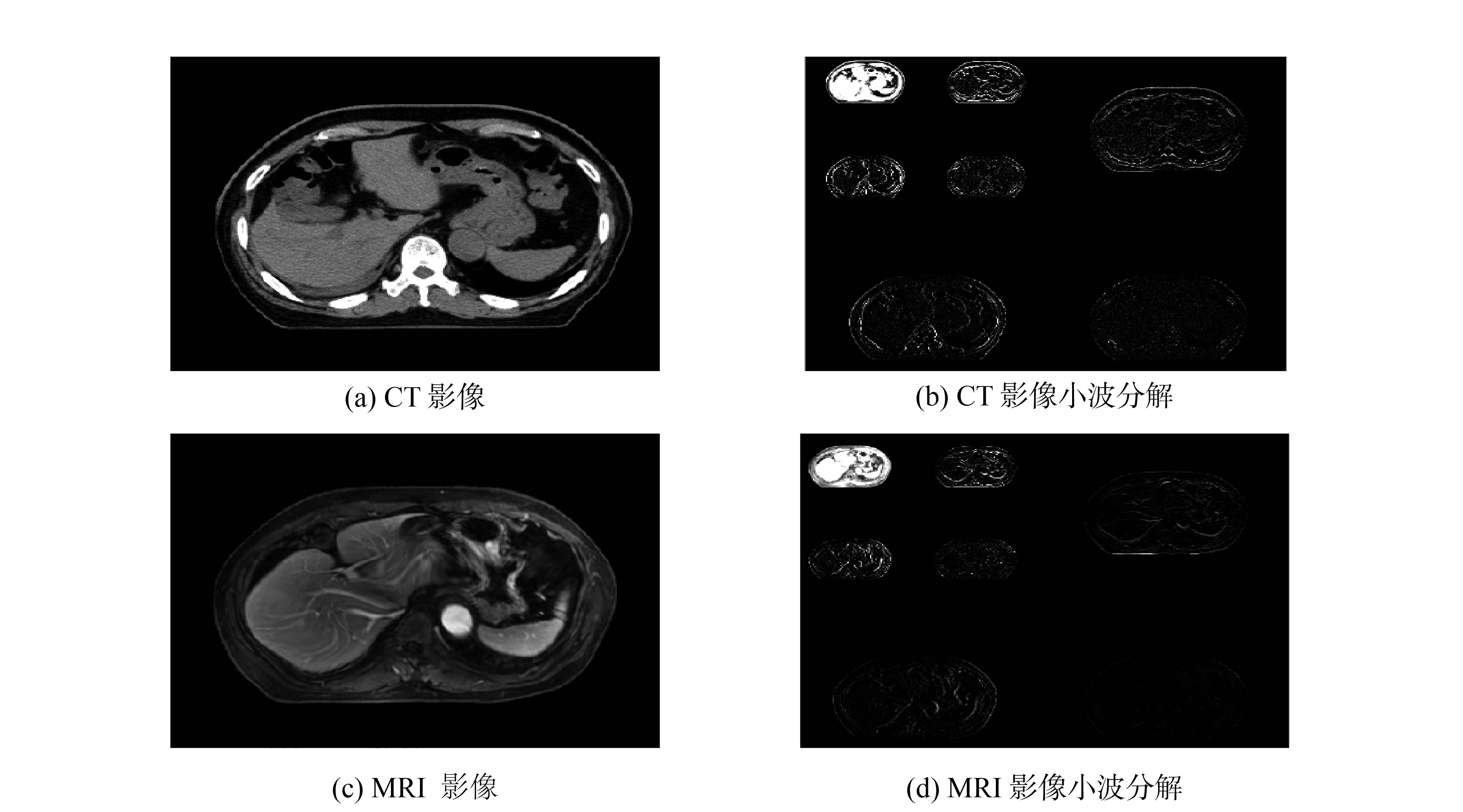Download: 图 10 小波分解结果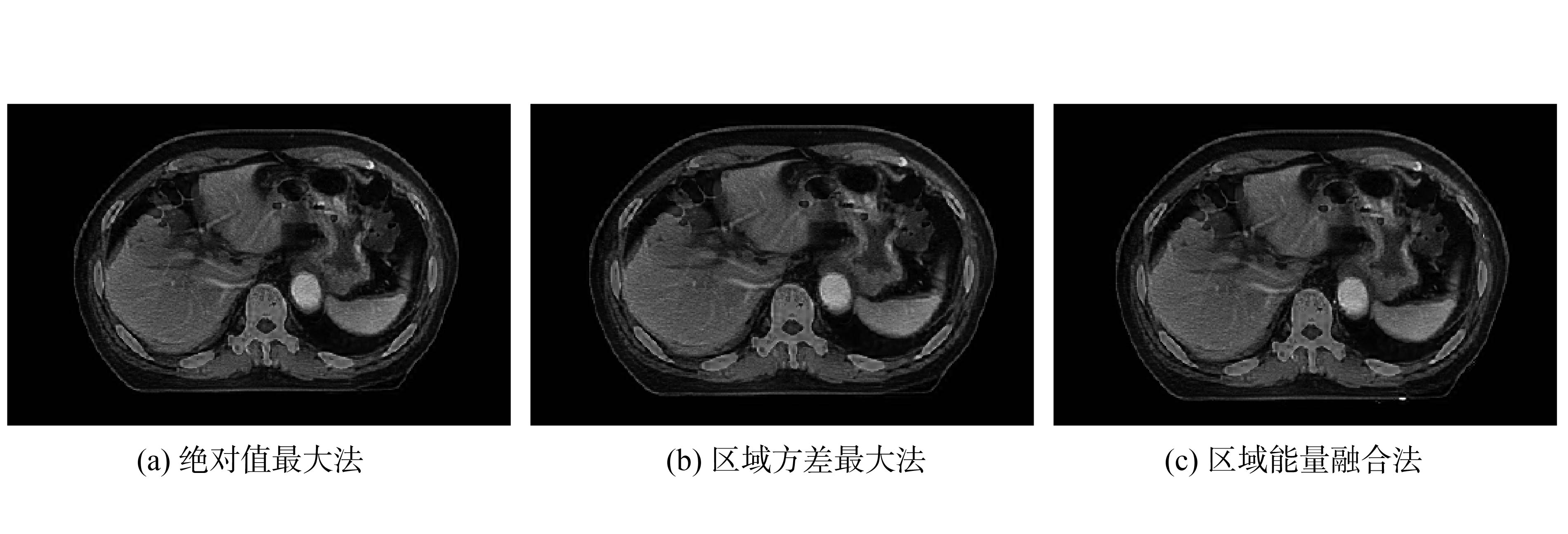Download: 图 11 影像融合结果表 1 不同融合方法的评价指标
3 结论

1)在影像配准部分采用基于仿射变换的粗配准与基于B样条变换的精配准相结合的非刚体配准方法，并用互信息测度评价配准程度，提高了配准的精度与计算速度。

2)在影像融合部分，提出在不同频带使用不同融合规则的基于小波变换的多分辨率融合方法，对低频系数进行加权平均融合，对高频系数分别采用绝对值最大法、区域方差最大法、区域能量融合法进行融合，融合结果最大限度地保留了多模态医学影像的有效信息。

  周涛, 陆惠玲. 医学数字图像处理[M]. 北京: 科学出版社, 2015: 26-35. (0)  于颖, 聂生东. 医学图像配准技术及其研究进展[J]. 中国医学物理学杂志, 2009, 26(6): 1485-1489. (0)  赵菲, 廖琪梅, 马昂昂, 等. 基于ITK的多模态医学图像非刚性配准研究[J]. 医疗卫生装备, 2011, 32(11): 5-8. DOI:10.3969/j.issn.1003-8868.2011.11.002 (0)  汪军. 基于B样条和互信息的非刚性医学图像配准的研究与应用[D]. 太原: 太原理工大学, 2017. (0)  赵涓涓, 屈明月, 强彦, 等. 基于互信息的肺部PET-CT图像非刚性配准[J]. 清华大学学报(自然科学版), 2013, 53(8): 1091-1097. (0)  聂秀玲, 刘任远, 陆加明, 等. 基于ITK多图谱配准的脑部皮质下核团的分割研究[J]. 中国生物医学工程学报, 2017, 36(5): 529-535. DOI:10.3969/j.issn.0258-8021.2017.05.003 (0)  UNSER M, ALDROUBI A, EDEN M. Fast B-spline transforms for continuous image representation and interpolation[J]. IEEE transactions on pattern analysis and machine intelligence, 1991, 13(3): 277-285. DOI:10.1109/34.75515 (0)  KYBIC J, UNSER M. Fast parametric elastic image registration[J]. IEEE transactions on image processing, 2003, 12(11): 1427-1442. DOI:10.1109/TIP.2003.813139 (0)  安绿波, 肖孟强. 基于B样条自由形变模型的医学图像非刚性配准算法研究[J]. 电脑知识与技术, 2012, 8(7): 1573-1575. DOI:10.3969/j.issn.1009-3044.2012.07.036 (0)  ZHU W, WANG Y, DENG Y, et al. A wavelet-based multiresolution regularized least squares reconstruction approach for optical tomography[J]. IEEE transactions on medical imaging, 1997, 16(2): 210-217. DOI:10.1109/42.563666 (0)  徐磊. 基于离散小波变换的多模态医学图像融合算法研究[D]. 南京: 南京医科大学, 2016. (0)  李美丽. 像素级图像融合算法与应用[M]. 西安: 西安电子科技大学出版社, 2016: 29-30. (0)  MALLAT S, ZHONG Sifen. Characterization of signals from multiscale edges[J]. IEEE transactions on pattern analysis and machine intelligence, 1992, 14(7): 710-732. DOI:10.1109/34.142909 (0)  马军红. 基于多小波变换的区域方差图像融合方法[J]. 光电子技术, 2012, 32(2): 99-104. DOI:10.3969/j.issn.1005-488X.2012.02.006 (0)  王阳萍, 杜晓刚, 赵庶旭, 等. 医学影像图像处理[M]. 北京: 清华大学出版社, 2012: 165-168. (0)  杨娇. 基于小波变换的图像融合算法的研究[D]. 北京: 中国地质大学(北京), 2014. (0)